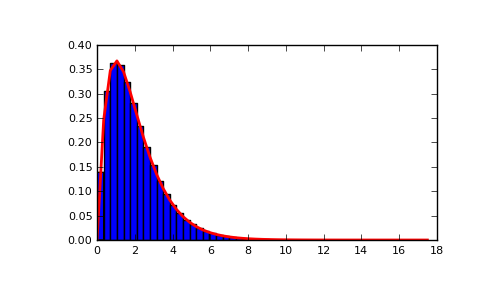numpy.random.RandomState.standard_gamma¶

RandomState.standard_gamma(shape, size=None)

Draw samples from a Standard Gamma distribution.

Samples are drawn from a Gamma distribution with specified parameters, shape (sometimes designated “k”) and scale=1.

Parameters : shape : float Parameter, should be > 0. size : int or tuple of ints Output shape. If the given shape is, e.g., (m, n, k), then m * n * k samples are drawn. samples : ndarray or scalar The drawn samples.

scipy.stats.distributions.gamma
probability density function, distribution or cumulative density function, etc.

Notes

The probability density for the Gamma distribution is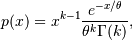where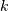is the shape and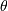the scale, and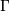is the Gamma function.

The Gamma distribution is often used to model the times to failure of electronic components, and arises naturally in processes for which the waiting times between Poisson distributed events are relevant.

References

 [R171] Weisstein, Eric W. “Gamma Distribution.” From MathWorld–A Wolfram Web Resource. http://mathworld.wolfram.com/GammaDistribution.html
 [R172] Wikipedia, “Gamma-distribution”, http://en.wikipedia.org/wiki/Gamma-distribution

Examples

Draw samples from the distribution:

>>> shape, scale = 2., 1. # mean and width
>>> s = np.random.standard_gamma(shape, 1000000)

Display the histogram of the samples, along with the probability density function:

>>> import matplotlib.pyplot as plt
>>> import scipy.special as sps
>>> count, bins, ignored = plt.hist(s, 50, normed=True)
>>> y = bins**(shape-1) * ((np.exp(-bins/scale))/ \
...                       (sps.gamma(shape) * scale**shape))
>>> plt.plot(bins, y, linewidth=2, color='r')
>>> plt.show()